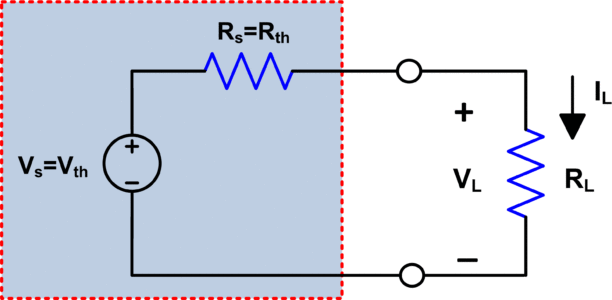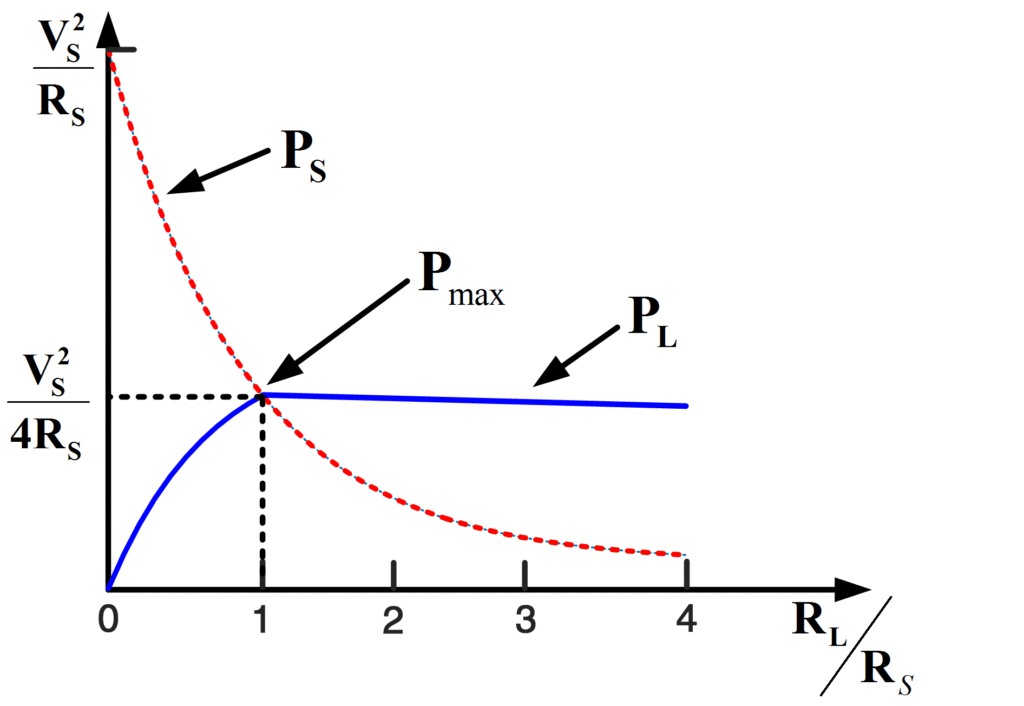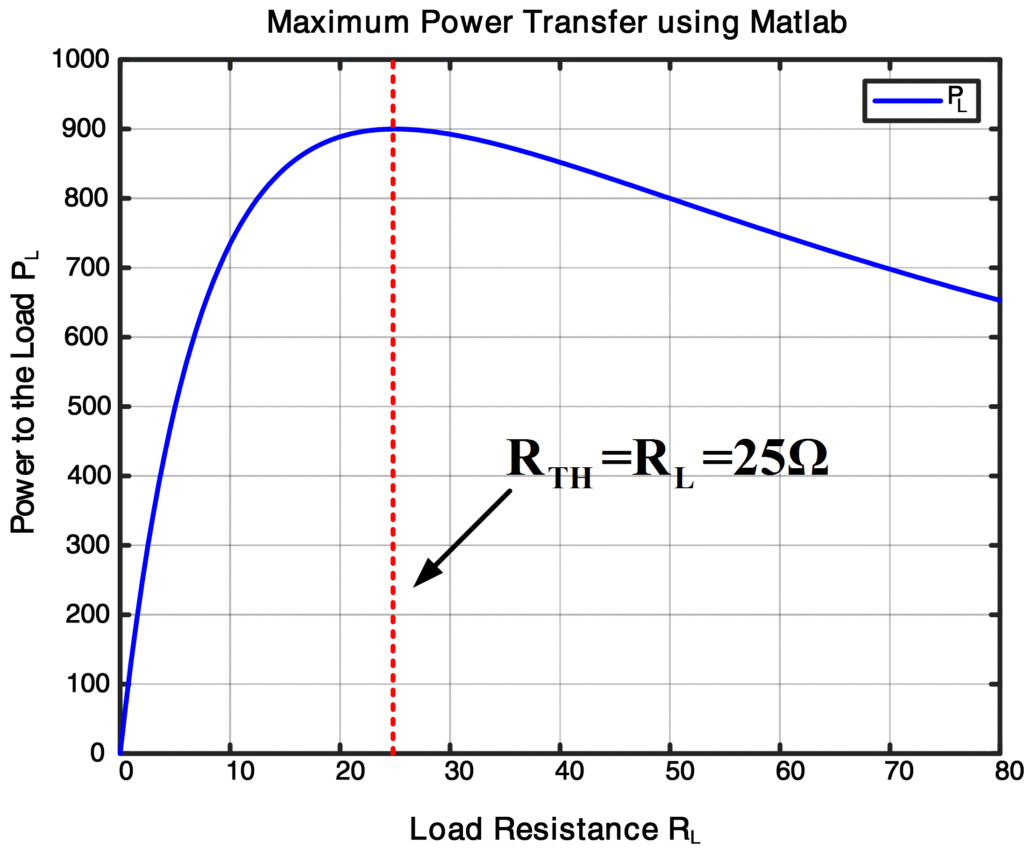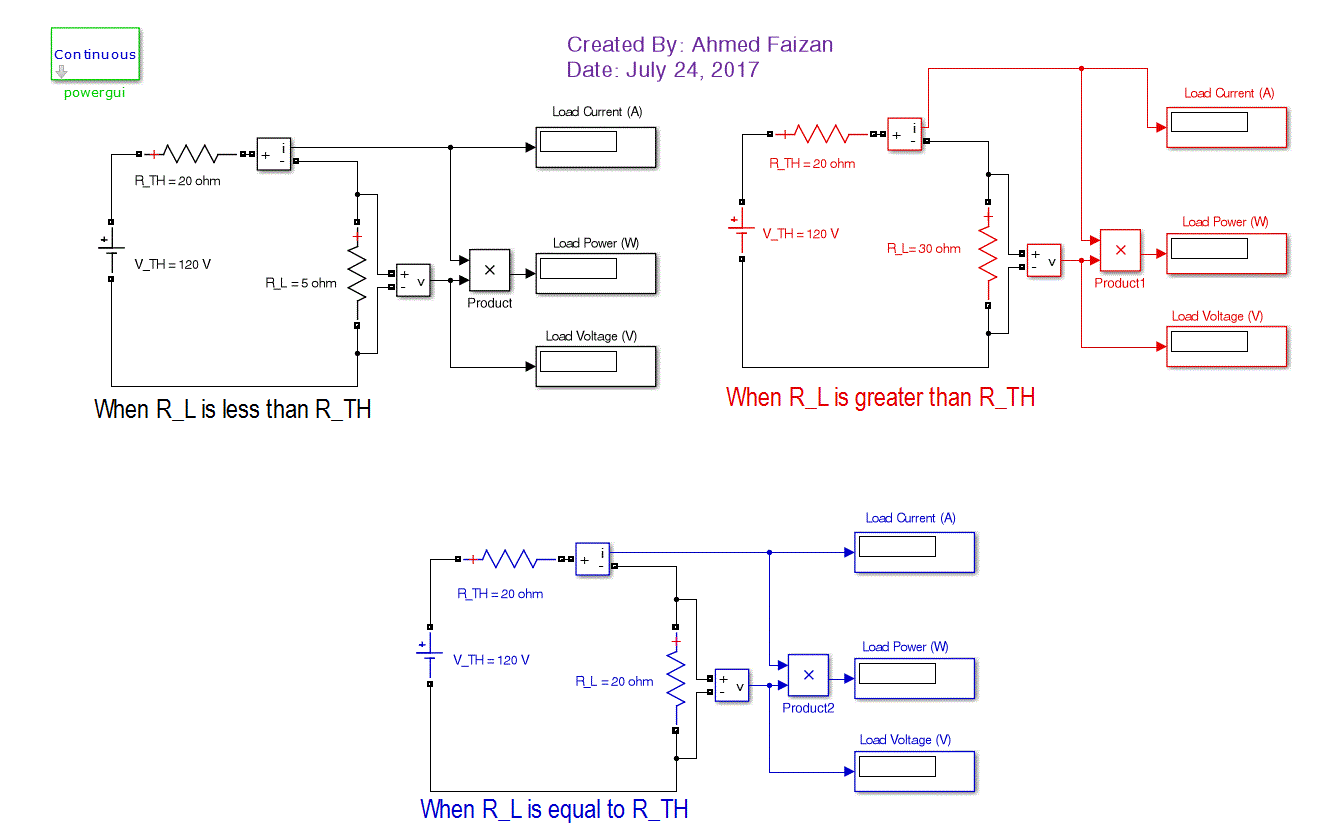Home / Basic Electrical / Maximum Power Transfer Theorem

# Maximum Power Transfer Theorem

Want create site? Find Free WordPress Themes and plugins.

## Maximum Power Transfer Theorem Definition

Maximum power transfer theorem states that maximum power output is obtained when the load resistance RL is equal to Thevenin resistance Rth as seen from load Terminals.Fig.1: Maximum Power Transfer Theorem

Any circuit or network may be represented by a Thevenin equivalent circuit.  The Thevenin resistance Rth is comparable to a source internal resistance (RS) which absorbs some of the power available from the ideal voltage source. In above figure, a variable load resistance RL is connected to a Thevenin circuit. The current for any value of load resistance RL is connected to a Thevenin circuit. The current for any value of load resistance is;

$I_L=\frac{{{V}_{S}}}{{{R}_{S}}+{{R}_{L}}}$

Then by using I2R , the power delivered to the load is,

${{P}_{L}}={{\left( \frac{{{V}_{S}}}{{{R}_{S}}+{{R}_{L}}} \right)}^{2}}{{R}_{L}}~\text{ }\cdots ~~~~~~~\left( 1 \right)$

The load power depends on both Rth (RS) and RL; however, Rth (RS) is considered constant for any particular network. Then one might get an idea of how PL varies with a change in RL by assuming values for Thevenin circuit of above figure and, in turn, calculating PL for different values of  RL.

## Maximum Power Transfer Theorem Derivation

As we know power delivered to load is,

${{P}_{L}}={{\left( \frac{{{V}_{S}}}{{{R}_{S}}+{{R}_{L}}} \right)}^{2}}{{R}_{L}}$

Taking a derivative on both sides;

$\frac{d{{P}_{L}}}{d{{R}_{L}}}=\frac{V_{S}^{2}{{\left( {{R}_{S}}+{{R}_{L}} \right)}^{2}}-2{{R}_{L}}\left( {{R}_{S}}+{{R}_{L}} \right)}{{{\left( {{R}_{S}}+{{R}_{L}} \right)}^{4}}}$

For PL to be maximum;

$\frac{d{{P}_{L}}}{d{{R}_{L}}}=0$

So,

$\frac{V_{S}^{2}\left( {{R}_{S}}-{{R}_{L}} \right)}{{{\left( {{R}_{S}}+{{R}_{L}} \right)}^{3}}}=0$

Finally,

${{R}_{S}}={{R}_{L}}$

So maximum power transferred is;

${{P}_{max}}=\frac{V_{S}^{2}}{4{{R}_{S}}}$

We got above expression by substituting RS=RL into equation (1).

## Maximum Power Transfer and Efficiency of Transmission

We observe that power transfer from a real source always produces ohmic heating in the source resistance. Calculations of such internal effects require information about the internal structure and cannot, in general, be based upon Thevenin or Norton equivalent networks. However, the entire load current iL usually passes through the internal resistance of a real source, so we represent the internal conditions by lumped parameters as shown in figure 1. The resulting internal power dissipated by RTH or RS is then

${{P}_{S}}={{R}_{S}}I_{L}^{2}=\frac{{{R}_{S}}}{{{({{R}_{S}}+{{R}_{L}})}^{2}}}*V_{S}^{2}$

The dashed curve in figure 2 shows that PS steadily decreases as RL increases and that PS=PL when RL/RS=1.Fig.2: Maximum Power Transfer and Transmission Efficiency

Since the total power generated by the source is PL+PS, the wasted internal power PS should be small compared to PL for efficient operation. Formally, we define the power-transfer efficiency as

$Efficiency=\frac{{{P}_{L}}}{{{P}_{L}}+{{P}_{S}}}$

Which is often expressed as a percentage. If the load has been matched for maximum power transfer, then PS=PL, and so efficiency,

$Efficiency=\frac{{{P}_{L}}}{2{{P}_{L}}}=50\text{ %}$

Moreover, with RL=RS, the terminal voltage drops to V=VTH/2. Clearly, electrical utilities would not, and should not, strive for maximum power transfer. Instead, they seek higher power-transfer efficiency by making PS as small as possible.

Maximum Power Transfer Solved ExampleFind RL

Solution

Let’s find Vth first across 150 Ω resistance

${{V}_{th}}={{V}_{S}}=360*\frac{150}{150+30}$

${{V}_{th}}={{V}_{S}}=300~V$

To find Rth or RS, short circuit the voltage source

${{R}_{th}}={{R}_{S}}=150~||~30=25\Omega$

So, for maximum power transfer, we know that

${{R}_{L}}={{R}_{th}}=25\text{ }\Omega$

Now, Find Maximum power transfer to the load

${{P}_{max}}=\frac{V_{S}^{2}}{4{{R}_{S}}}=900~W$

## Maximum Power Transfer Theorem using Matlab Code

Here is the MATLAB code to implement maximum power transfer theorem in Matlab.


clear all;close all;clc
%% Circuit Parameters as given in the example (text)
% Matlab Code for Maximum Power Transfer Theorem
V_TH = 300; % Thevenin's Voltage
R_TH = 25; % Thevenin's Equivalent Resistance
R_L = 0:0.5:80; % Load Resistance
%%
%% Load Current &amp;amp;amp; Power Calculation
IL = V_TH./(R_TH + R_L); % Load Current
P_L = IL.^2 .* R_L; % Load Power
%%
% As we know that maximum power transfer occurs when R_TH=R_L
%% Plotting the Results
plot(R_L,P_L,'b')
hold on
title('Maximum Power Transfer using Matlab');
xlabel('Load Resistance R_L');
ylabel('Power to the Load P_L');
gtext('R_TH = R_L = 25 Ohm')
legend('P_L')
grid on



Result

Here is a graph which clearly shows that maximum power transfer occurs when Rth=RL.## Maximum Power Transfer Theorem using Matlab Simulink

Here, a Matlab Simulink model has been developed for three different cases:

1. When RL>RTH
2. When RL=RTH
3. When RL<RTHTo download and run the model, Click Here.

## When do we want maximum power transfer?

Primarily, in applications where voltage and current signals are used to convey information rather than to deliver large amounts of power. For instance, the first stage of a radio or television receiver should get as much power as possible out of the information-bearing signals that arrive via antenna or cable. Those tiny signals account for only a small fraction of the total power consumed by the receiver, and so power-transfer efficiency is not a significant concern.

Did you find apk for android? You can find new Free Android Games and apps.

### About Ahmad FaizanMr. Ahmed Faizan Sheikh, M.Sc. (USA), Research Fellow (USA), a member of IEEE & CIGRE, is a Fulbright Alumnus and earned his Master’s Degree in Electrical and Power Engineering from Kansas State University, USA.